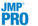Publication date: 07/30/2020

##SVD Report

The SVD option produces two SVD plots and a table of the singular values from the singular value decomposition.

###SVD Plots

The first plot contains a point for each transaction. For a given transaction, the point that is plotted is defined by the transaction’s values in the first two singular vectors (the first two columns of the U matrix) multiplied by the diagonal singular values matrix (S). This plot is equivalent to the Score Plot in the Principal Components platform. Each point in this plot represents a transaction. In the Transaction SVD plot, points that are visibly grouped together indicate transactions with a similar composition.

The second plot contains a point for each item. For a given item, the point that is plotted is defined by the item’s values in the first two singular vectors (the first two rows of the V matrix) multiplied by the diagonal singular values matrix (S). This plot is equivalent to the Loadings Plot in the Principal Components platform. Each point in this plot represents an item. In the Item SVD plot, items that are visibly grouped together indicate items that have similar functions or topic areas.

###Singular Values

Below the transaction and item SVD plots, a table of the singular values appears. These are the diagonal entries of the S matrix in the singular value decomposition of the transaction item matrix. The Singular Values table also contains a column of corresponding eigenvalues for the equivalent principal components analysis. Like in the Principal Components platform, there are columns for the percent and cumulative percent of variation explained by each eigenvalue (or singular value). You can use the Cum Percent column to decide what percent of variance from the transaction item matrix you want to preserve, and then use the corresponding number of singular vectors.